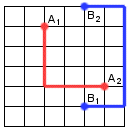### Problem Statement

The problem statement contains an image.

In VLSI design it's important to connect certain pairs of points on a circuit using as little wire as possible. Given an empty circuit board of size N x N, we want to connect the two points A1 and A2 with each other using one wire, and the two points B1 and B2 with each other using another wire. The wires must go along the horizontal and vertical edges on the grid (see figure), and the two wires may not share a common vertex.Create a class ConnectingPoints containing the method shortestWires, which takes an int N, the number of vertices on one side of the circuit board, and a pair of int[], X and Y, each containing four elements. Elements 0 and 1 in X and Y contain the coordinates for A1 and A2, and elements 2 and 3 in X and Y contain the coordinates for B1 and B2. The method should return the minimum length of wires needed to connect A1 with A2 and B1 with B2. If it's not possible to connect the points, return -1 (see example 1).

### Definition

 Class: ConnectingPoints Method: shortestWires Parameters: int, int[], int[] Returns: int Method signature: int shortestWires(int N, int[] X, int[] Y) (be sure your method is public)

### Constraints

-N will be between 2 and 500, inclusive.
-X and Y will each contain exactly four elements.
-Each element in X and Y will be between 0 and N-1, inclusive.
-All four coordinate pairs described by X and Y will be distinct.

### Examples

0)

 `7` `{2,5,4,4}` `{1,4,5,0}`
`Returns: 15`
 This is the example in the problem statement.
1)

 `100` `{0,99,0,99}` `{0,99,99,0}`
`Returns: -1`
 The points to be connected are on the opposite corners of the circuit board, and there is no way to connect them with each other without crossing wires. Hence the method returns -1.
2)

 `500` `{250,251,2,495}` `{273,273,273,273}`
`Returns: 496`
3)

 `13` `{5,5,5,5}` `{3,7,6,11}`
`Returns: 13`
4)

 `5` `{2,4,4,3}` `{3,0,2,0}`
`Returns: 16`

#### Problem url:

http://www.topcoder.com/stat?c=problem_statement&pm=3978

#### Problem stats url:

http://www.topcoder.com/tc?module=ProblemDetail&rd=6553&pm=3978

Yarin

#### Testers:

PabloGilberto , lbackstrom , vorthys

#### Problem categories:

Brute Force, Greedy, Search